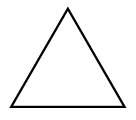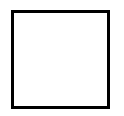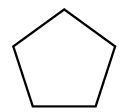### Home > CC1MN > Chapter 1 Unit 1 > Lesson CC1: 1.1.2 > Problem1-12

1-12.

Consider the first three figures of the pattern below.Figure 1Figure 2Figure 3

1. On your own paper, draw what Figure 4 of this pattern should look like.

How many sides are in each figure shown?
How many would you expect in the next figure?

2. Using words, describe what Figures 5 and 6 should look like.

If Figure 4 has 6 sides of the same length, how many sides should the next figures have?

Figure 5 should have seven sides of the same length.
Figure 6 should have eight sides of the same length.

3. Using words, describe how the pattern is changing.

• How is one figure different from the previous one?
Think about how you answered part (b) and try to generalize this.